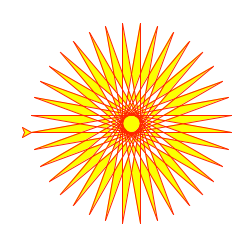# Graphics

Wave provides a versatile API for rendering vector graphics (SVG) backed by data buffers.

Use the graphics card ui.graphics_card() to render graphics.

## Stages and scenes#

The graphics card has two attributes that control how graphics are rendered: `stage` and `scene`:

• A stage holds static shapes that are rendered as part of the background (literally, behind the scene).
• A scene holds shapes whose attributes need to be animated (causing a re-render, or, literally, cause a scene).

Use `ui.stage()` and `ui.scene()` to create the stage and the scene:

• `ui.stage()` creates a packed, static representation of its shapes (recall that the stage is not supposed to change).
• `ui.scene()` declares a data buffer to efficiently update its shapes if changed (recall that the scene is animated).

Here's an example that draws a clock with a circular `face` placed on the `stage`, and `hour`, `min` and `sec` hands placed in the `scene`:

``````from h2o_wave import site, ui, graphics as g
page = site['/demo']page['example'] = ui.graphics_card(    box='1 1 2 3', view_box='0 0 100 100', width='100%', height='100%',    stage=g.stage(        face=g.circle(cx='50', cy='50', r='45', fill='#111', stroke_width='2px', stroke='#f55'),    ),    scene=g.scene(        hour=g.rect(x='47.5', y='12.5', width='5', height='40', rx='2.5', fill='#333', stroke='#555'),        min=g.rect(x='48.5', y='12.5', width='3', height='40', rx='2', fill='#333', stroke='#555'),        sec=g.line(x1='50', y1='50', x2='50', y2='16', stroke='#f55', stroke_width='1px'),    ),)``````

## Shapes#

Use drawing functions to add shapes to the stage or scene:

PrimitiveUse
arc()Circular or annular sector, as in a pie or donut chart
circle()Circle
ellipse()Ellipse
image()Image
line()Line
path()Path
polygon()Polygon
polyline()Polyline
spline()Curves (including radial curves) using various algorithms: basis, cardinal, smooth, linear, monotone, natural, step.
rect()Rect
text()Text

## Paths#

The `path()` function is special: it can draw arbitrary shapes. This is because SVG path elements support an attribute called `d` which holds drawing commands.

Here's a path that represents a red square:

``g.path(d='m 25 25 h 50 v 50 h -50 z', fill='red')``

The above snippet produces:

``<path d='m 25 25 h 50 v 50 h -50 z' fill='red'/>``

The drawing commands in the above example work like this:

1. `m 25 25`: Move 25px left, 25px down
2. `h 50`: Draw a line 50px right
3. `v 50`: Draw a line 50px down
4. `h -50`: Draw a line 50px left
5. `z`: Close the path (going back to where we started)

The little drawing syntax above is part of the SVG specification, not something unique to Wave.

Authoring `path()` drawing commands by hand is tedious, so Wave provides two utilities to make it easier: `p()` and `turtle()`.

## Drawing with paths#

`p()` creates a path generator with convenience methods to draw step by step. For example, the following two lines are equivalent:

``red_square = g.path(d='m 25 25 h 50 v 50 h -50 z', fill='red')red_square = g.p().m(25, 25).h(50).v(50).h(-50).z().path(fill='red')``

### Commands#

MethodUse
`M()`Move, absolute
`H()`Horizontal line, absolute
`V()`Vertical line, absolute
`L()`Line, absolute
`A()`Elliptical arc, absolute
`C()`Cubic Bézier curve, absolute
`S()`Cubic Bézier curve, smooth, absolute
`Q()`Quadratic Bézier curve, absolute
`T()`Quadratic Bézier curve, smooth, absolute
`Z()`Close path
`m()`Move, relative
`h()`Horizontal line, relative
`v()`Vertical line, relative
`l()`Line, relative
`a()`Elliptical arc, relative
`c()`Cubic Bézier curve, relative
`s()`Cubic Bézier curve, smooth, relative
`q()`Quadratic Bézier curve, relative
`t()`Quadratic Bézier curve, smooth, relative
`z()`Close path
`d()`Serialize this path's commands into SVG path data.
`path()`Create a SVG path element
##### info

The upper-cased commands use absolute coordinates. The lower-cased commands use relative coordinates. For example, `L(4,2)` means "draw a line to (4,2)", but `l(4,2)` means "draw a line 4px right, 2px down from the current position".

## Drawing with a turtle#

`turtle()` creates a path generator (similar to `p()`), but using Turtle Geometry.

### Commands#

MethodUse
`pd()`Pen down
`pu()`Pen up
`p()`Set the turtle's position
`a()`Set the turtle's orientation
`f()`Move forward
`b()`Move backward
`l()`Turn left
`r()`Turn right
`d()`Serialize this turtle's movements into SVG path data
`path()`Create a SVG path element

### Example#

Here is an example from Python's turtle module:Here is the above example recreated in Wave:

``from turtle import *color('red', 'yellow')begin_fill()while True:    forward(200)    left(170)    if abs(pos()) < 1:        breakend_fill()done()``
``````from h2o_wave import site, ui, graphics as g
t = g.turtle().f(100).r(90).pd()for _ in range(36):    t.f(200).l(170)spirograph = t.pu(1).path(stroke='red', fill='yellow')
page = site['/demo']page['example'] = ui.graphics_card(    box='1 1 2 3', view_box='0 0 220 220', width='100%', height='100%',    scene=g.scene(foo=spirograph),)
page.save()``````
##### info

Turtle graphics is not just kid stuff: See Turtle Geometry by Harold Abelson and Andrea diSessa.